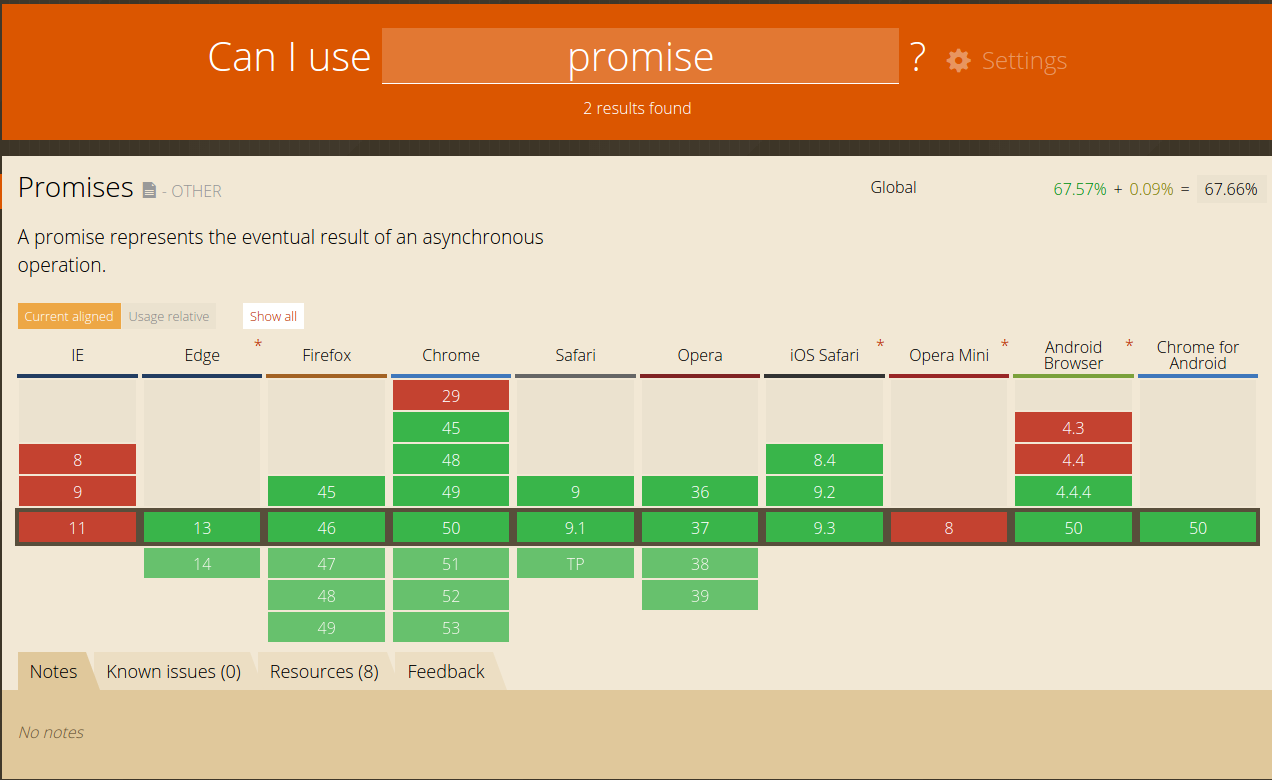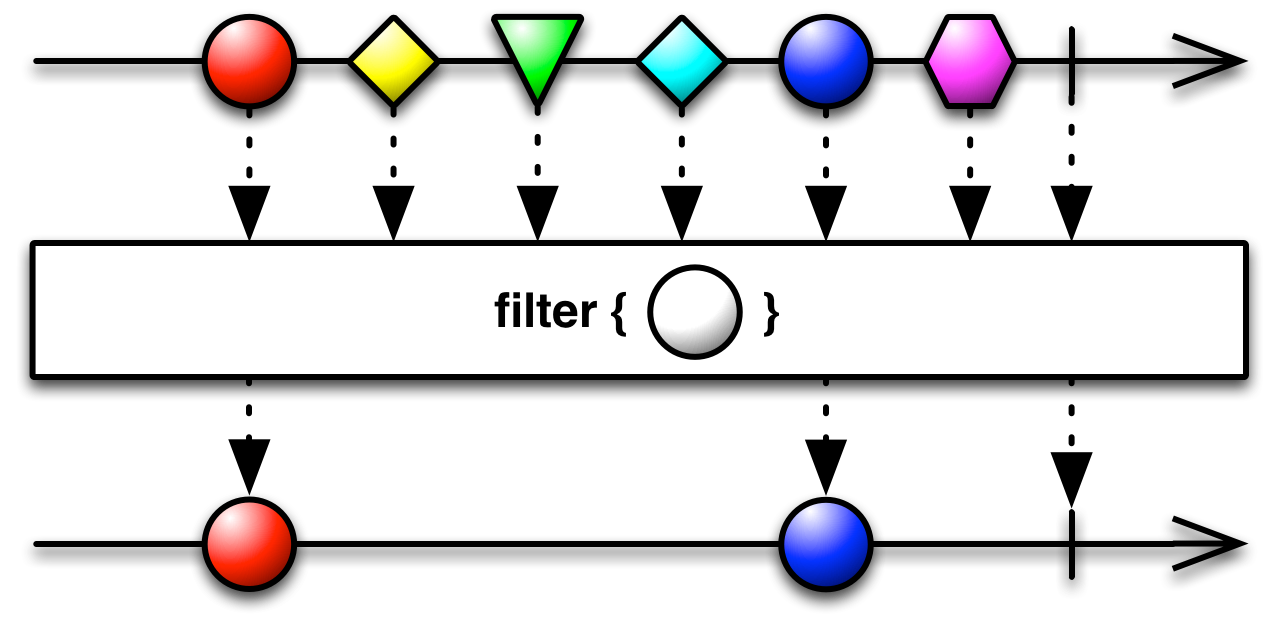# Promise vs Observable

Posted by YanYang Yu on May 16, 2016

# Promise/A+

Promise通过链式调用让我们能够以同步的形式编写异步流程，当然了要多写一些额外的代码，例如

``````promise.then(getData).then(getNextData).then(getNextNextData).catch(fail)...
``````## 延迟

``````var promise = new Promise((resolve,reject)=> {
console.log('promise exec');
resolve(5);
});
``````

``````function getProdList(){
return new Promise((resolve,reject)=> {
// http
});
}

getProdList().then(res => {
// handle res
});
``````

## 取消

``````function getProdList() {
return new Promise((resolve, reject)=> {
var xhr = new XMLHttpRequest();
if (xhr.status === 200) {
resolve(xhr.responseText);
} else {
reject(xhr.responseText);
}
}
};

xhr.open('GET', 'package.json', true);
xhr.send();
});
}

\$fetch.click(()=> {
getProdList().then(res => {
console.log(res);
});
});
``````

``````\$cancel.click(()=> {
// todo
});
``````

## 重试

``````function retry(fn, times) {
return fn().catch(err => {
if (times <= 0) return Promise.reject(err);
return retry(fn, times--);
});
}

retry(getProdList, 2);
``````

# Observable

RxJS（Reactive Extensions for javascript），响应式的思路是把随时间不断变化的数据、状态、事件等等转成可被观察的序列(Observable Sequence)，然后订阅序列中那些Observable对象的变化。Observable可以同我们数组操作进行类比，例如有这样一个数组

``````var arr = [1, 2, 3, 4];
``````

``````var newArr = arr.map(x => x * x).filter(x => x <= 10);
``````

``````Rx.Observable.from(arr)
.map(x => x * x)
.filter(x => x <= 10)
.subscribe(x => console.log(x));
``````

``````Rx.Observable.fromEvent(\$fetch, 'click')
.subscribe(e => console.log(e.target === \$fetch));
``````

## 延迟

``````var source = Rx.Observable.create(observer=> {
console.log('observable exec');
observer.onNext(5);
observer.onCompleted();
});
``````

``````source.subscribe(x => console.log(x));
``````

## 取消

``````var source = Rx.Observable.create(observer=> {
var xhr = new XMLHttpRequest();
if (xhr.status === 200) {
observer.onNext(xhr.responseText);
} else {
observer.onError(new Error(xhr.responseText));
}
observer.onCompleted();
}
};

xhr.open('GET', 'package.json', true);
xhr.send();

return function () {
xhr.abort();
}
});

var subscription;

Rx.Observable.fromEvent(\$fetch, 'click')
.subscribe(e => {
subscription = source.subscribe(
res => console.log(res),
err => console.log(err)
);
});

Rx.Observable.fromEvent(\$cancel, 'click')
.subscribe(e => {
if (subscription) {
subscription.dispose();
}
});
``````

## 重试

``````var source = Rx.Observable.create(observer=> {
var xhr = new XMLHttpRequest();
if (xhr.status === 200) {
observer.onNext(xhr.responseText);
} else {
observer.onError(new Error(xhr.responseText));
}
observer.onCompleted();
}
};

xhr.open('GET', 'package.gjson', true);
xhr.send();

return function () {
xhr.abort();
}
}).retry(3);
``````

## 小结

Rx.Observable提供了丰富的API供我们使用，十分方便。但是有时候写法会比较复杂，例如事件绑定

``````Rx.Observable.fromEvent(\$fetch, 'click')
.subscribe(e => {

});
``````

RxJS是函数式编程的衍生物，所以我们要以函数式编程的思想去看待，不要想着什么OOP了。

# 函数式编程

``````function add(x, y){
return x + y;
}
``````

add函数就是一个纯函数，固定的输入获得固定的输出，如`add(1,2)`输出永远是3。

``````function random(x){
return x + Math.random();
}
``````

random函数就不是纯函数，因为输出是随机的。

## 组合

Observable的链式调用实际上运用了函数式编程当中的组合：通过函数的结合产生新的函数

``````var compose = function(f,g) {
return function(x) {
return f(g(x));
};
};
``````

``````var compose = function(e,f,g) {
return function(x) {
return e(f(g(x)));
};
};
``````

ramda是一个javascript函数式编程库，提供了如curry、compose等等方法。 上面先map后filter的例子利用ramda改成函数式写法

``````function pow2(x) {
return x * x;
}

function lt10(x) {
return x <= 10;
}

var resultFn = R.compose(R.filter(lt10), R.map(pow2));
resultFn(arr);
``````